基于树莓派的物联网河流流量计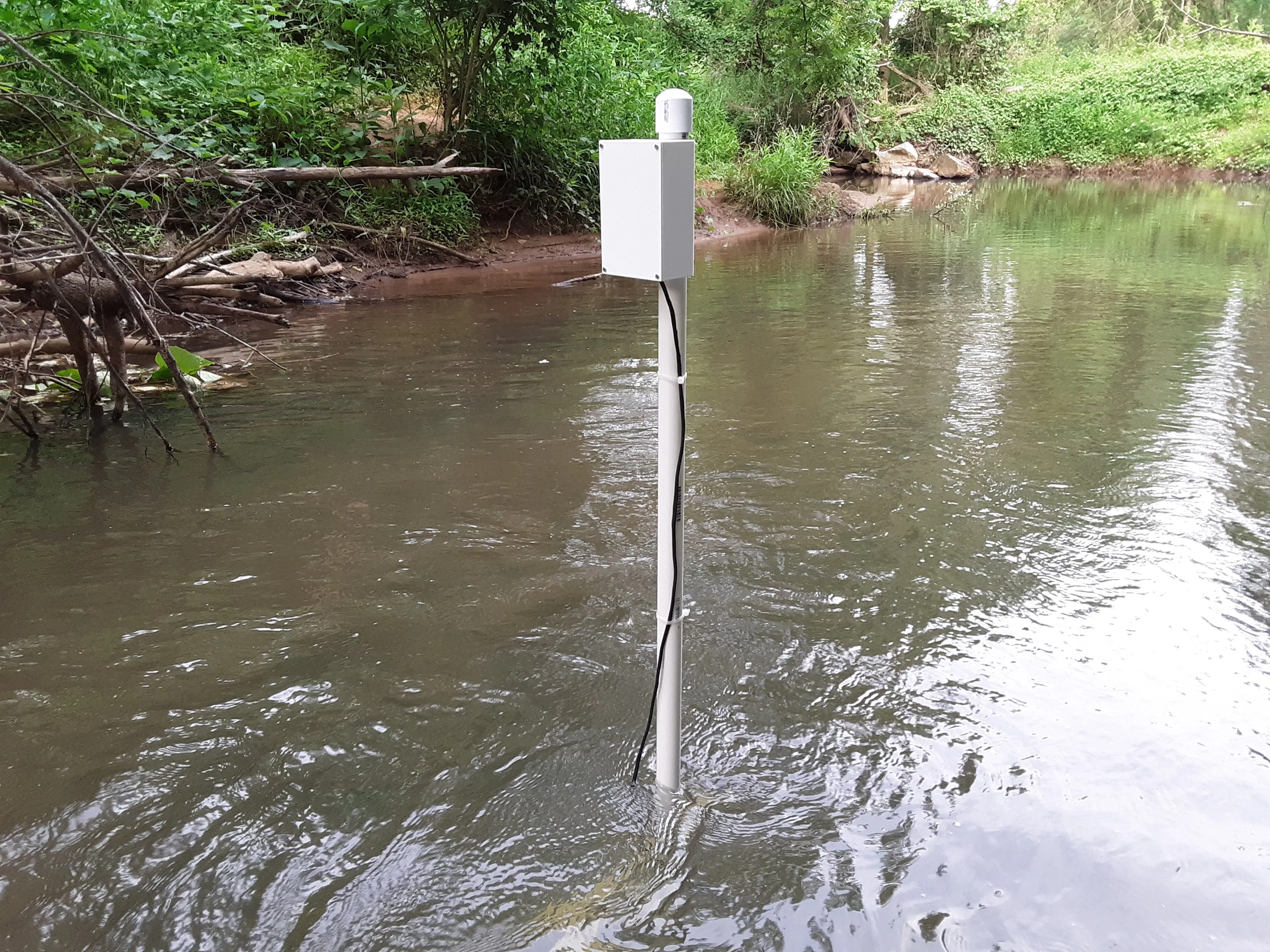• 水文：流速的长期趋势可以深入了解为河流供水的流域系统及其随时间的变化。
• 生态：河水的流速决定了它是否可以养活不同种类的植物或动物。
• 洪水预警：流量峰值可以为即将到来的洪水提供早期预警，并且可以与降雨报告相关联。
• 娱乐安全：实时测量可以警告独木舟/皮划艇运动员沿河旅行是否安全。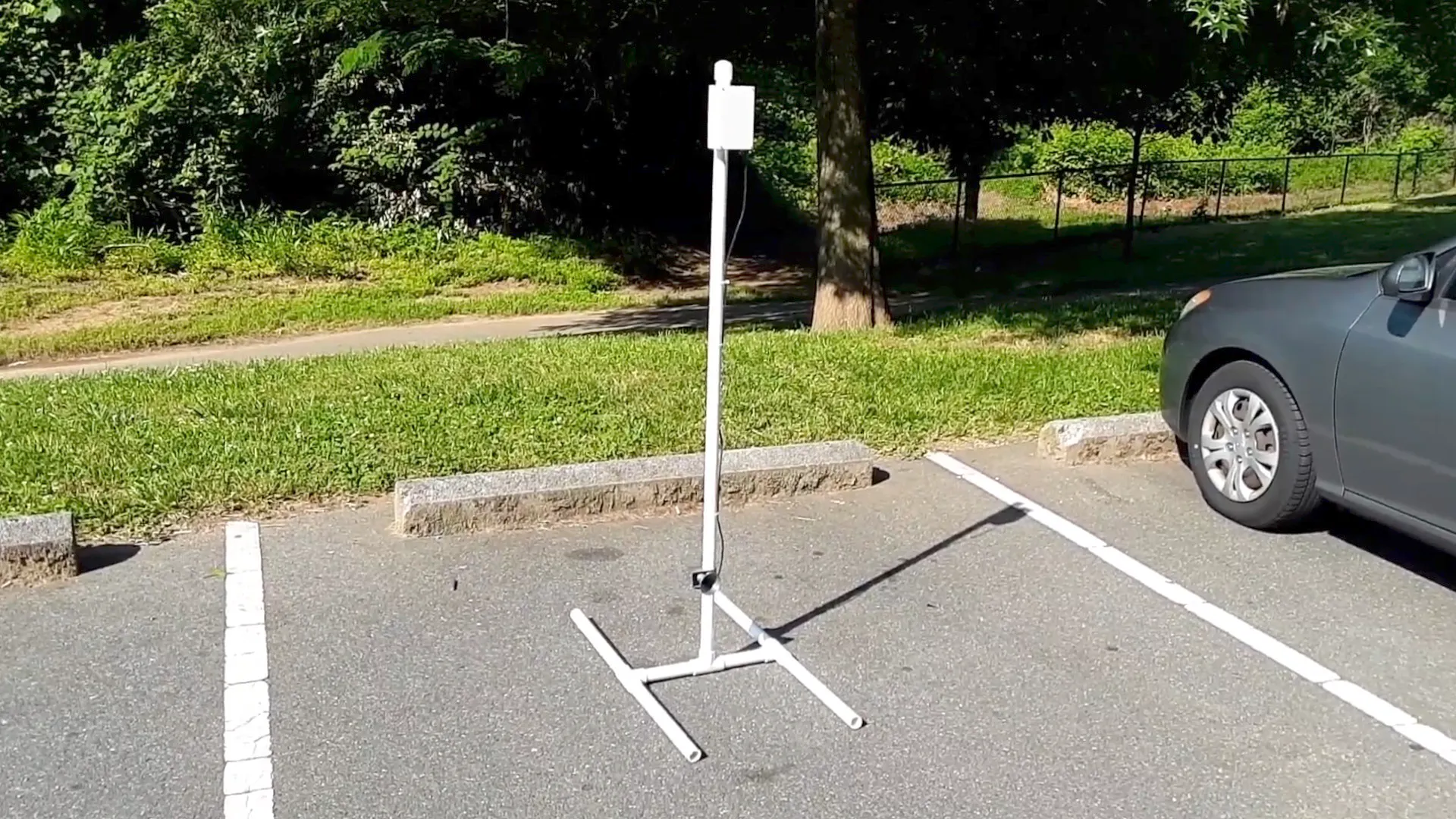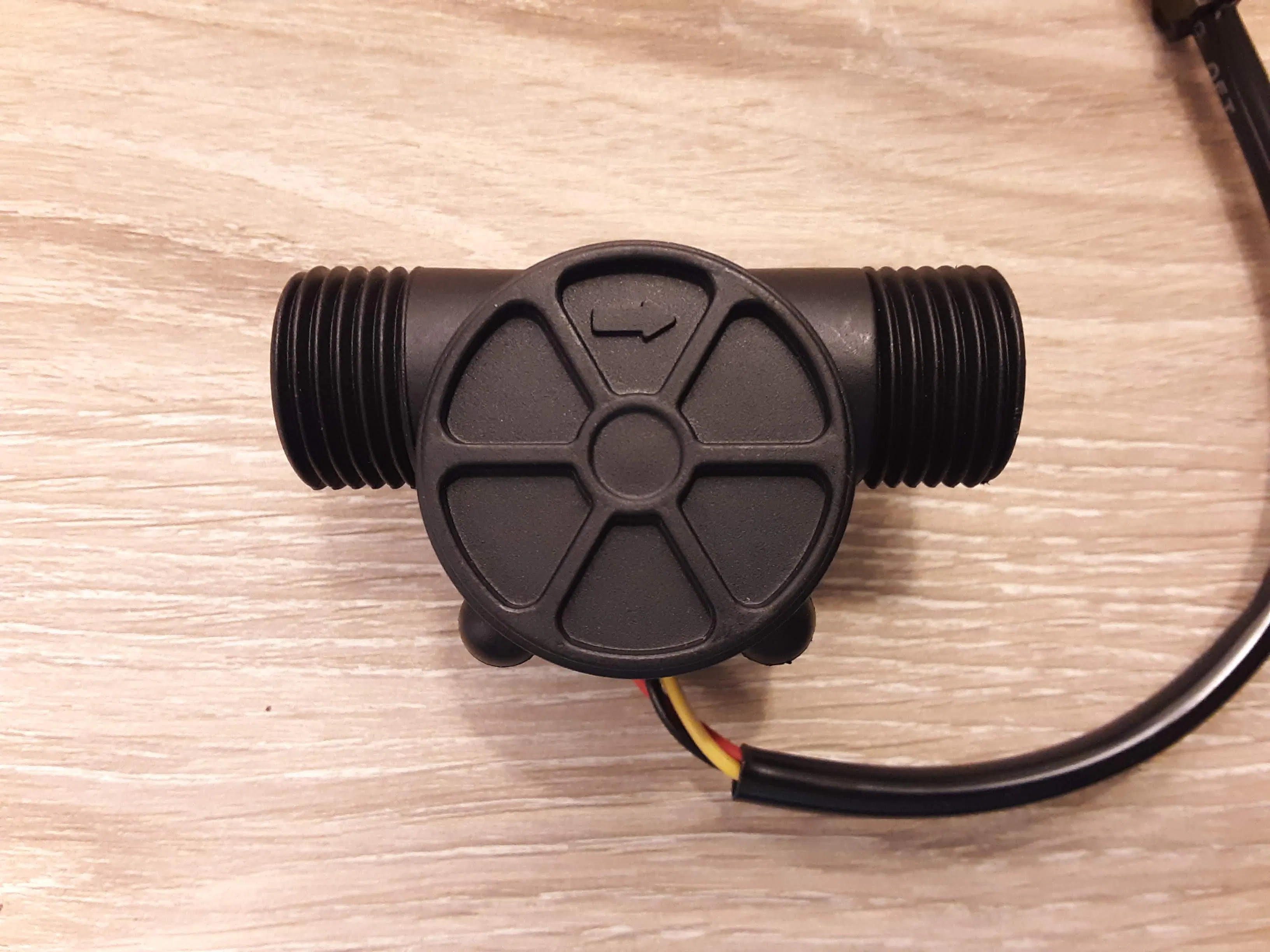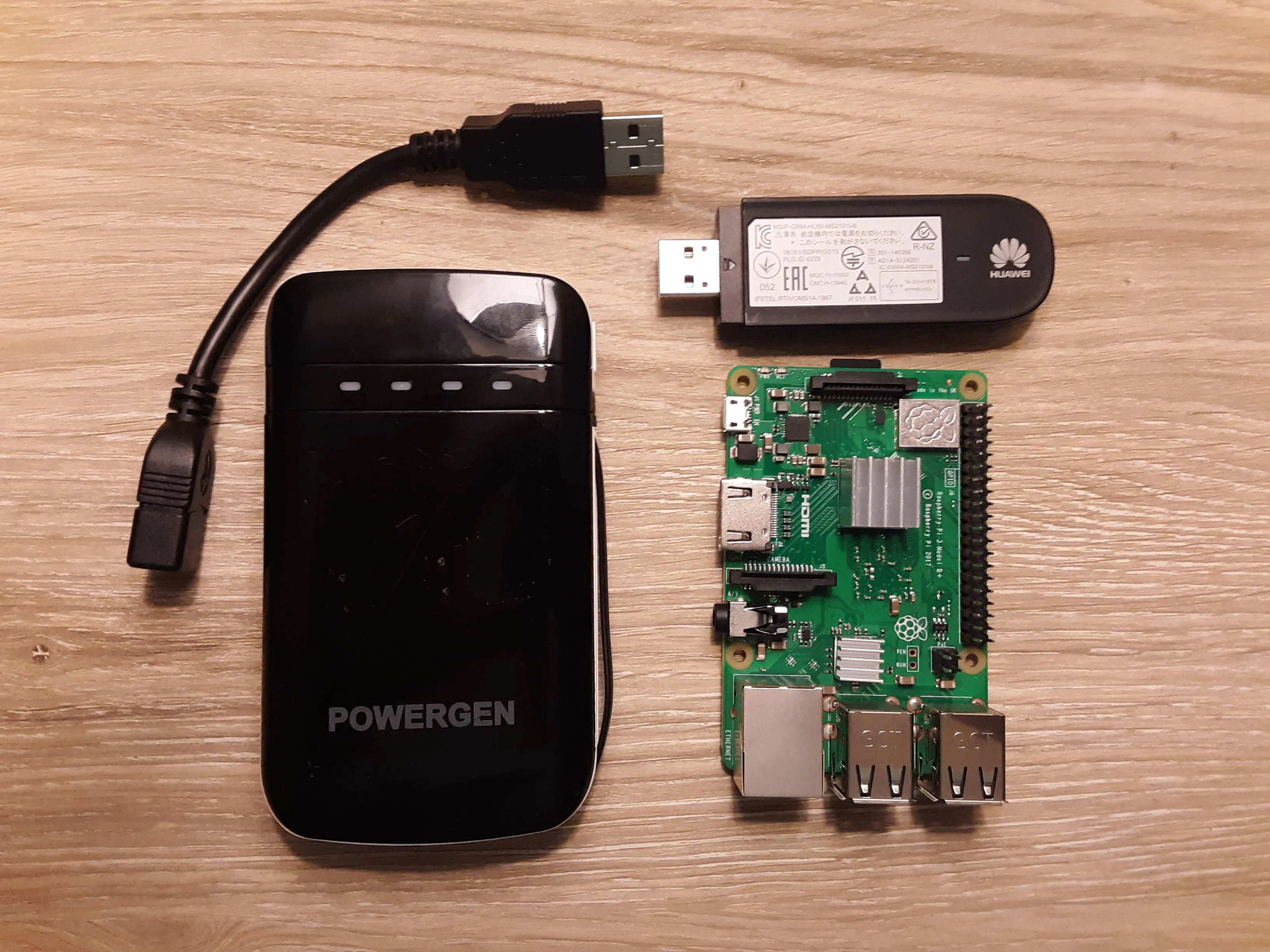Pi 运行 Python 脚本，每分钟都会从传感器收集流量测量值，并使用调制解调器和 SIM 卡提供的蜂窝连接将其推送到云端。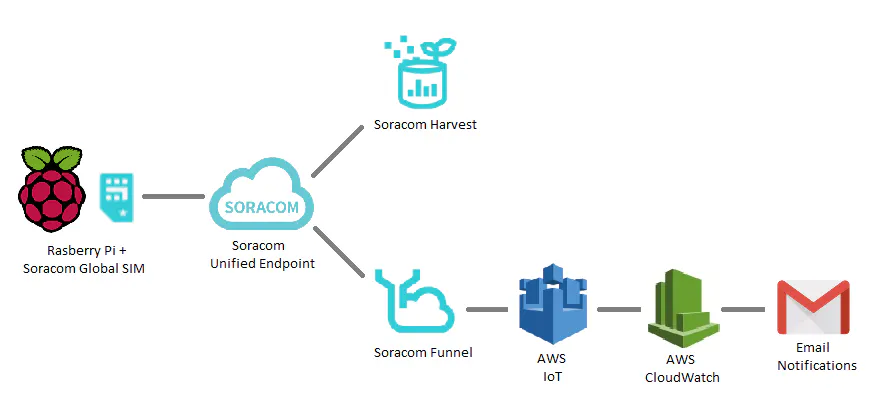Soracom Harvest 允许对传入数据进行轻松的实时可视化。控制台显示一个实时图表，显示更新的数据值，因为它们流入。用户可以监控数据在选定的时间窗口内如何变化。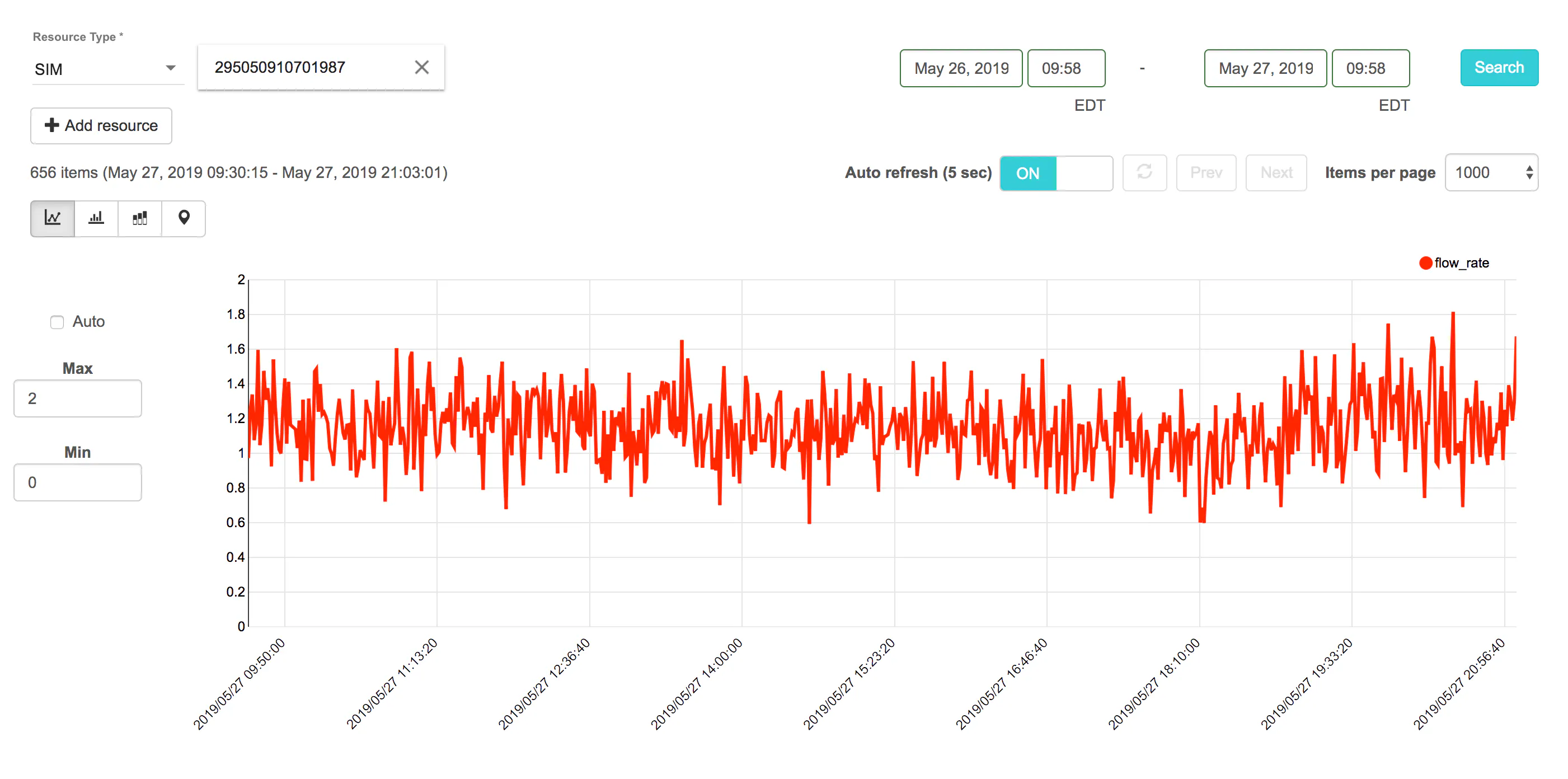Soracom Funnel 获取数据并通过将其发送到 AWS IoT 端点进一步转发，然后使用 AWS IoT 规则将数据推送到 AWS CloudWatch。

CloudWatch 警报配置为监控流量何时超过临界值，并自动向任何订阅用户发送电子邮件通知，让他们知道警报已被触发。此功能允许用户随时了解河流流量的重要变化，而无需实时监控。

• 红色（直流电源）到引脚 1 (3.3V)
• 黑色（接地）到引脚 6（接地）
• 黄色（输出）到引脚 7 (GPIO4)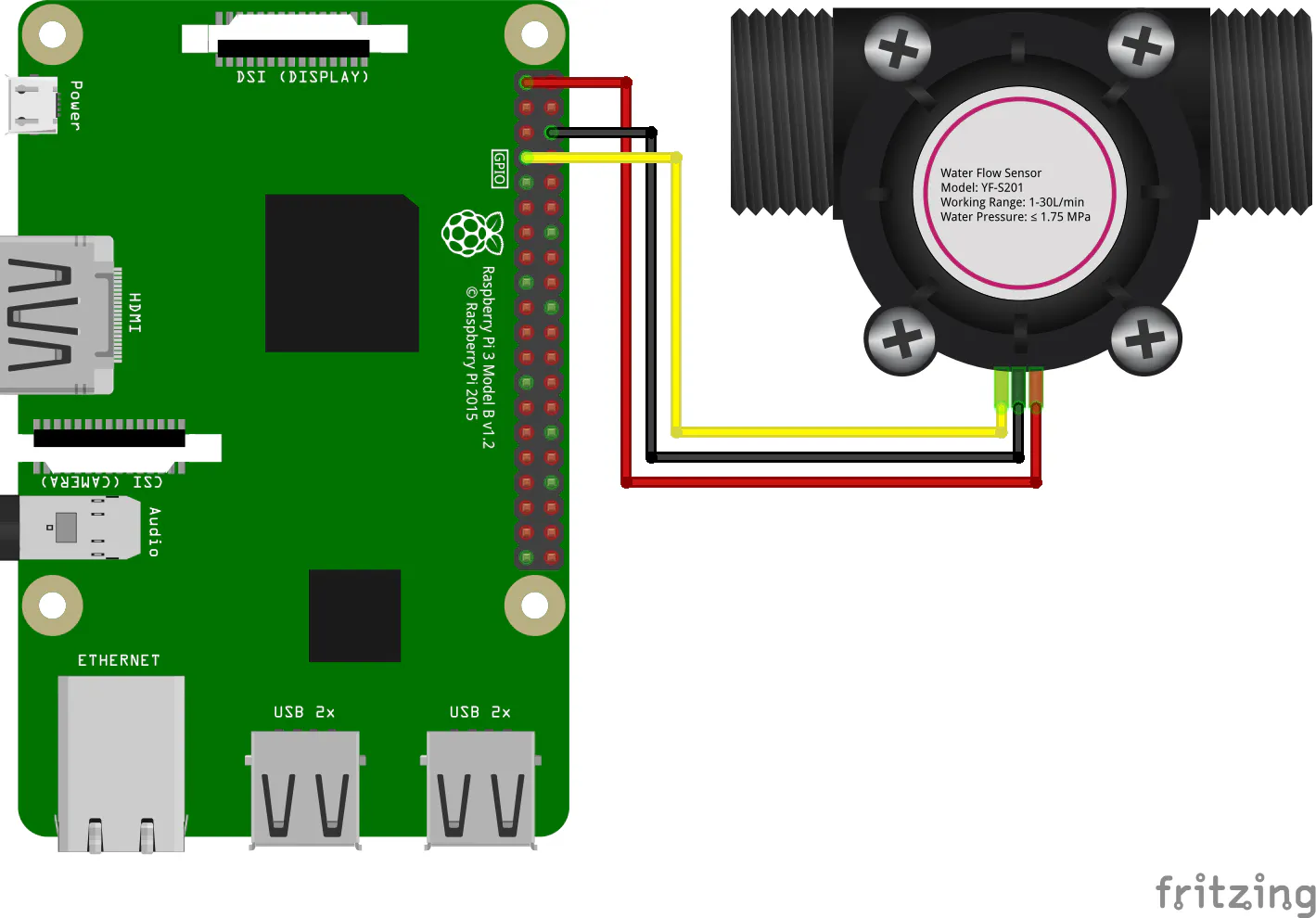import json
import time
from datetime import datetime
import RPi.GPIO as GPIO

class FlowMeter():
''' Class representing the flow meter sensor which handles input pulses
and calculates current flow rate (L/min) measurement
'''

def __init__(self):
self.flow_rate = 0.0
self.last_time = datetime.now()

def pulseCallback(self, p):
''' Callback that is executed with each pulse
'''

# Calculate the time difference since last pulse recieved
current_time = datetime.now()
diff = (current_time - self.last_time).total_seconds()

# Calculate current flow rate
hertz = 1. / diff
self.flow_rate = hertz / 7.5

# Reset time of last pulse
self.last_time = current_time

def getFlowRate(self):
''' Return the current flow rate measurement.
If a pulse has not been received in more than one second,
assume that flow has stopped and set flow rate to 0.0
'''

if (datetime.now() - self.last_time).total_seconds() > 1:
self.flow_rate = 0.0

return self.flow_rate

def main():
''' Main function for repeatedly collecting flow rate measurements
and sending them to the SORACOM API
'''

# Configure GPIO pins
INPUT_PIN = 7
GPIO.setmode(GPIO.BOARD)
GPIO.setup(INPUT_PIN, GPIO.IN, pull_up_down=GPIO.PUD_UP)

# Init FlowMeter instance and pulse callback
flow_meter = FlowMeter()
GPIO.RISING,
callback=flow_meter.pulseCallback,
bouncetime=20)

# Begin infinite loop
while True:

# Get current timestamp and flow meter reading
timestamp = str(datetime.now())
flow_rate = flow_meter.getFlowRate()
print('Timestamp: %s' % timestamp)
print('Flow rate: %f' % flow_rate)

# Delay
time.sleep(5)

if __name__ == '__main__':
main()Soracom 自己的IoT 状态机套件教程提供了有关使用 Soracom 帐户进行设置、配置 USB 调制解调器和启用 Harvest 服务的良好说明。从他们的教程中完成以下部分：main()在语句上方的函数内添加以下代码time.sleep(5)：flowmeter.py需要完整路径，因为 Pi 在flowmeter启动时不会在目录中。在行尾包含&确保脚本作为自己的进程运行，并且在运行时不会阻塞其他启动进程。

PVC 管结构将组件固定在适当的位置，使传感器保持在水下，电子设备保持在水面上。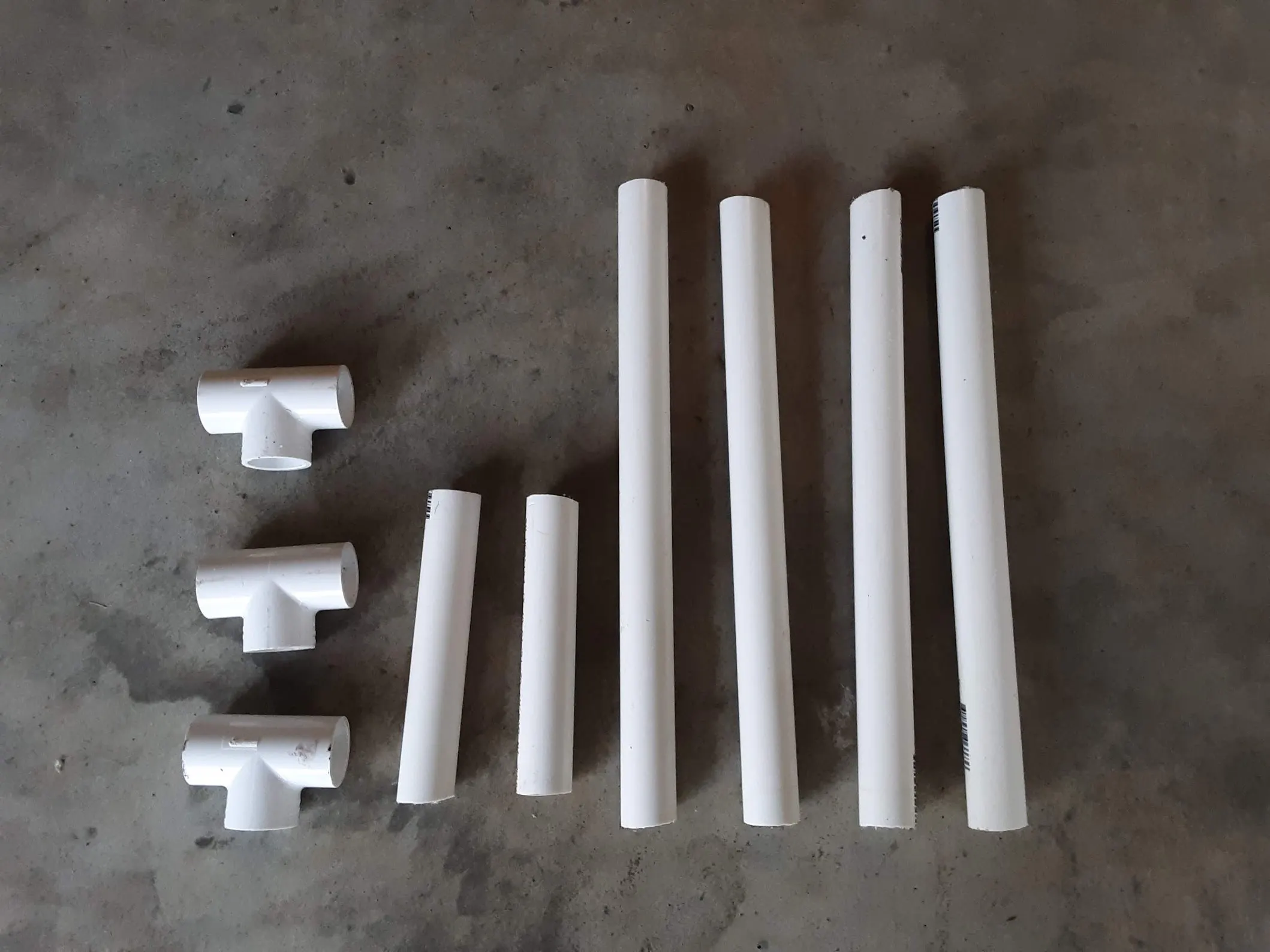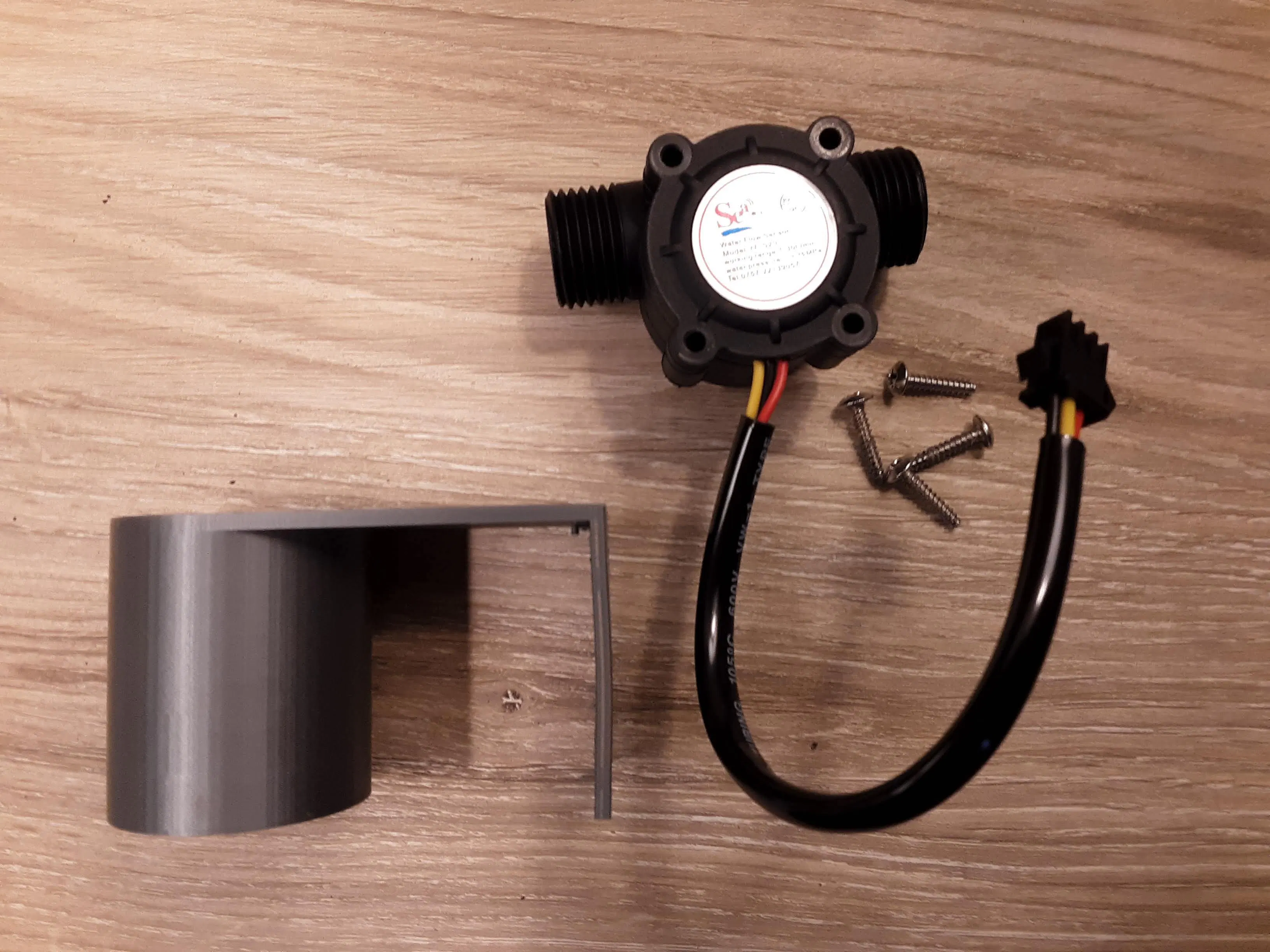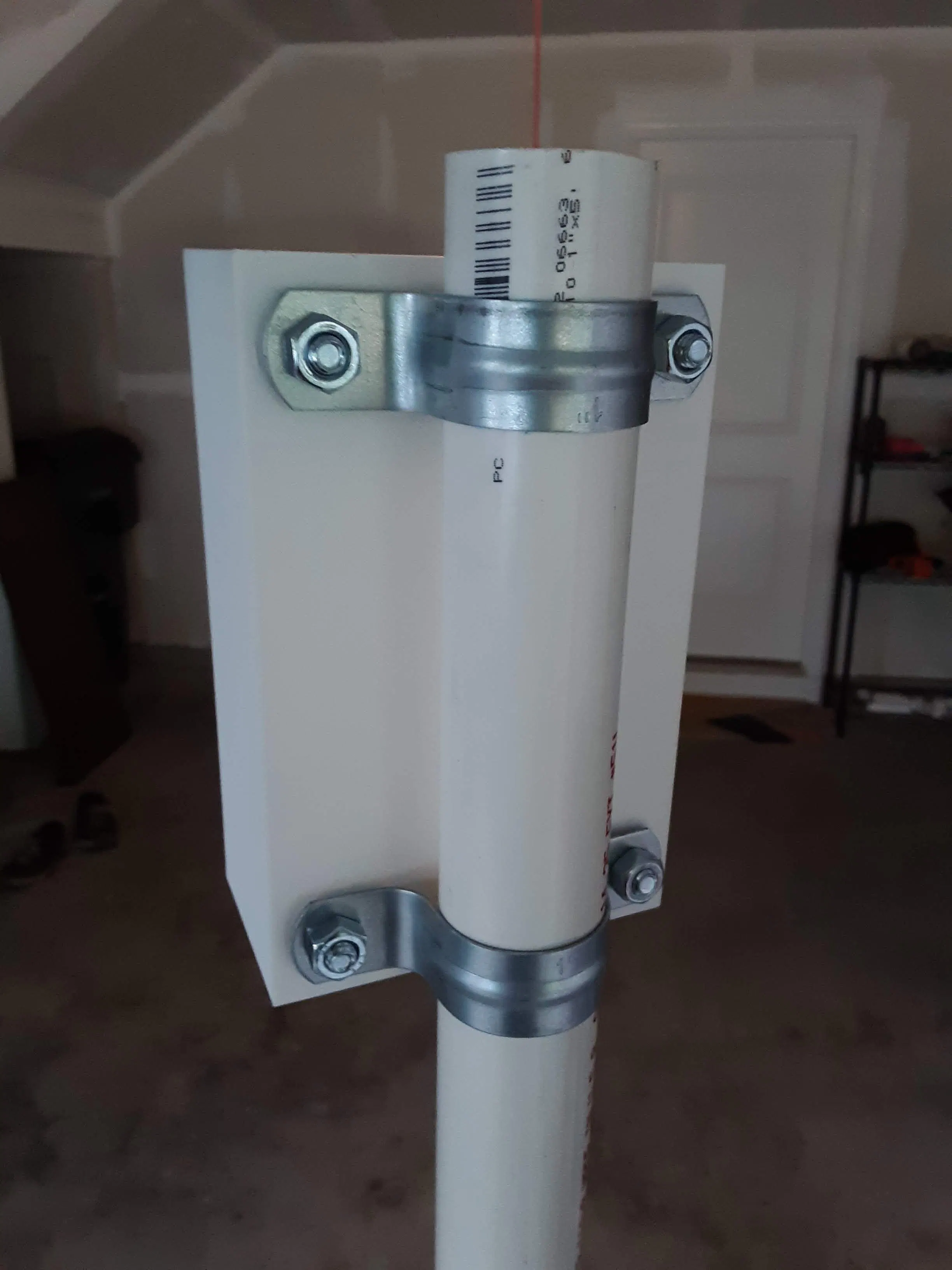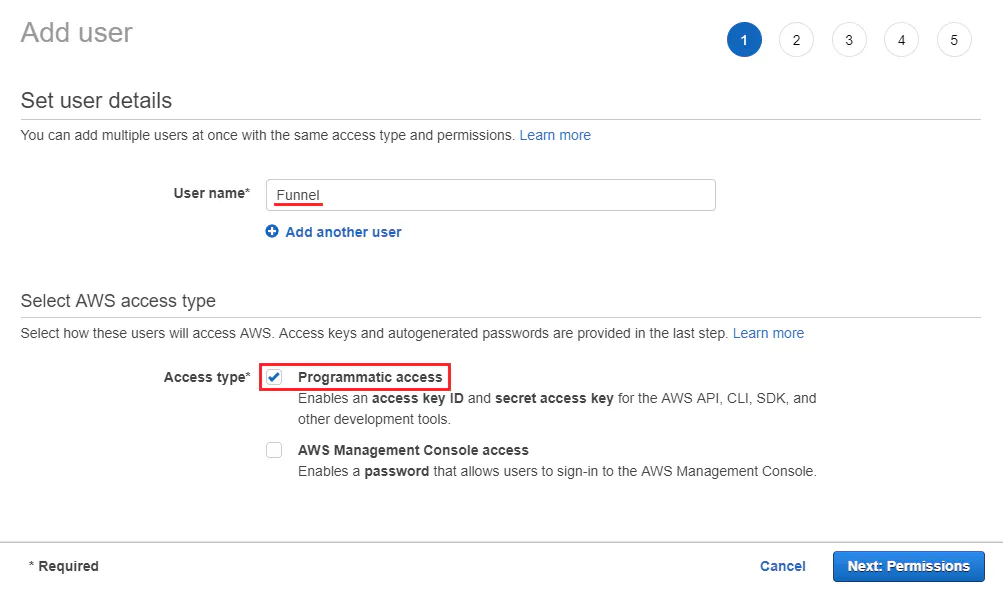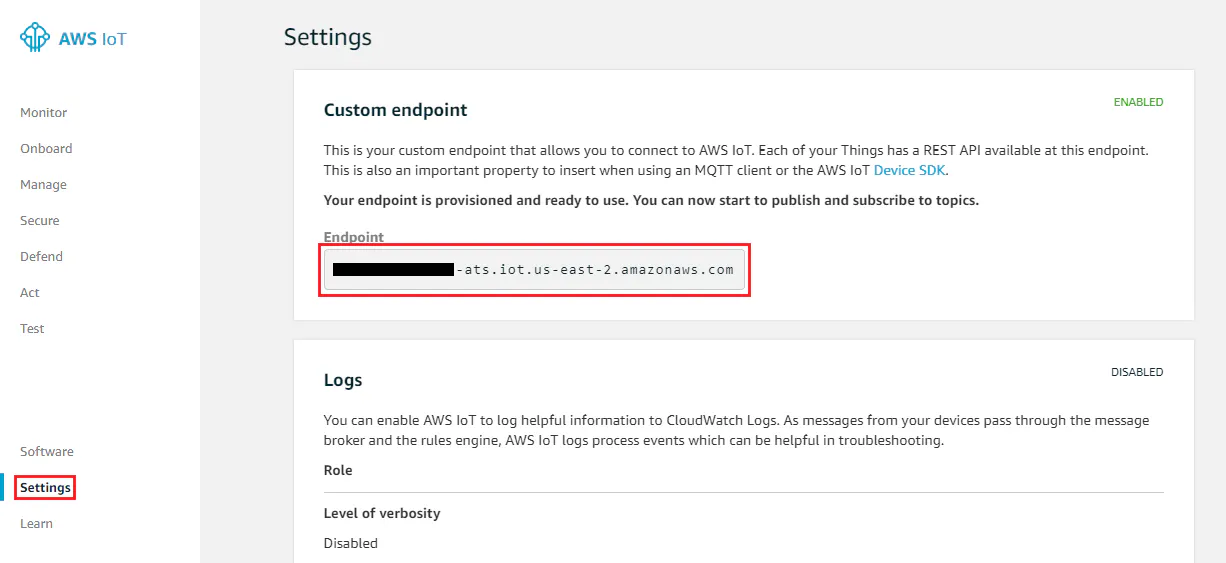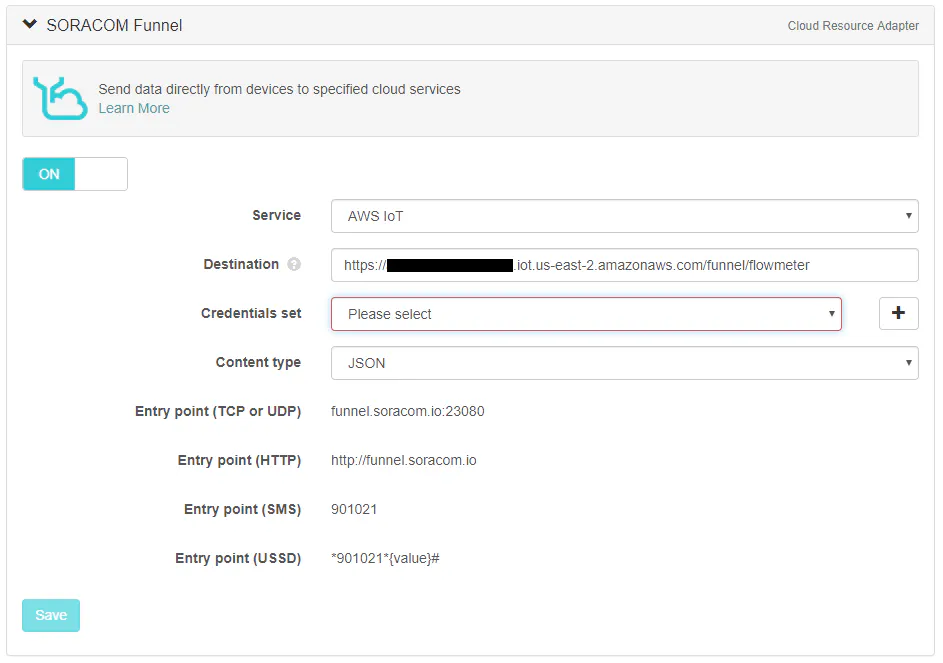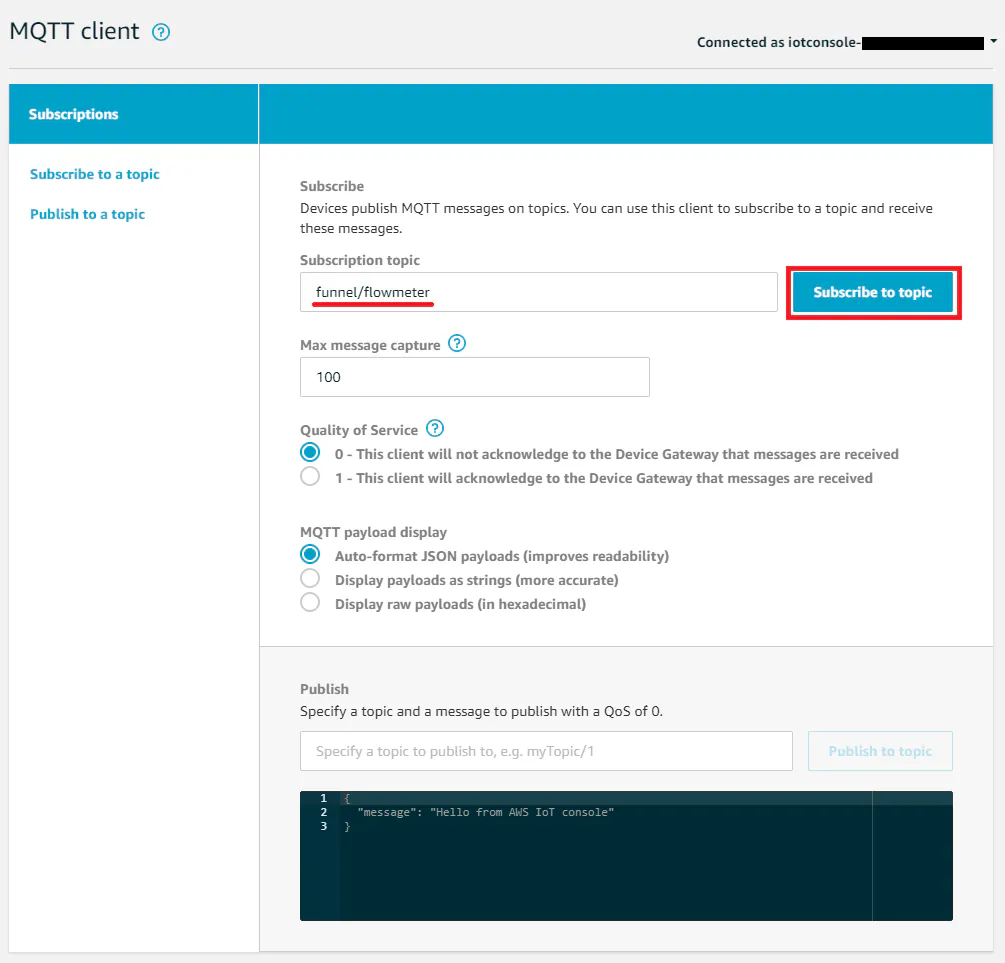• 指标名称： flow_rate
• 指标命名空间： flowmeter
• 单元： None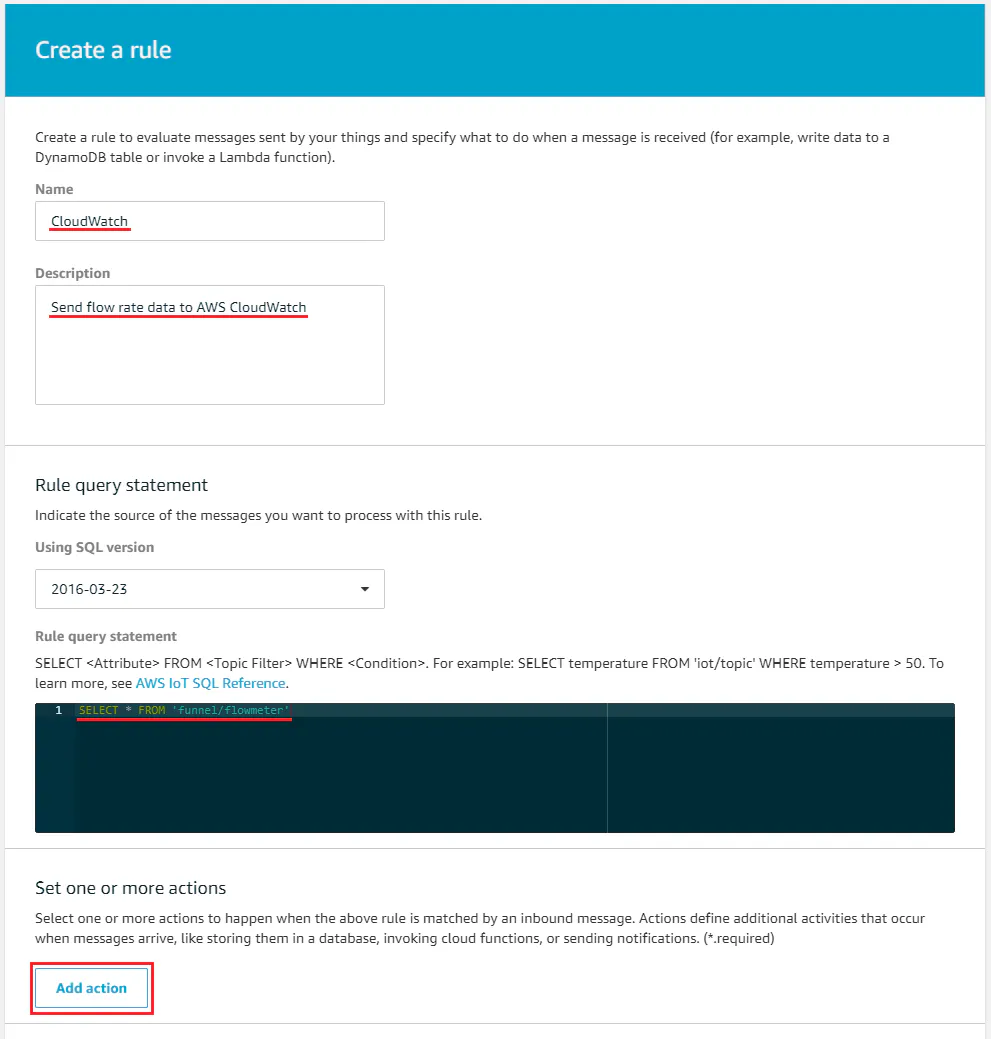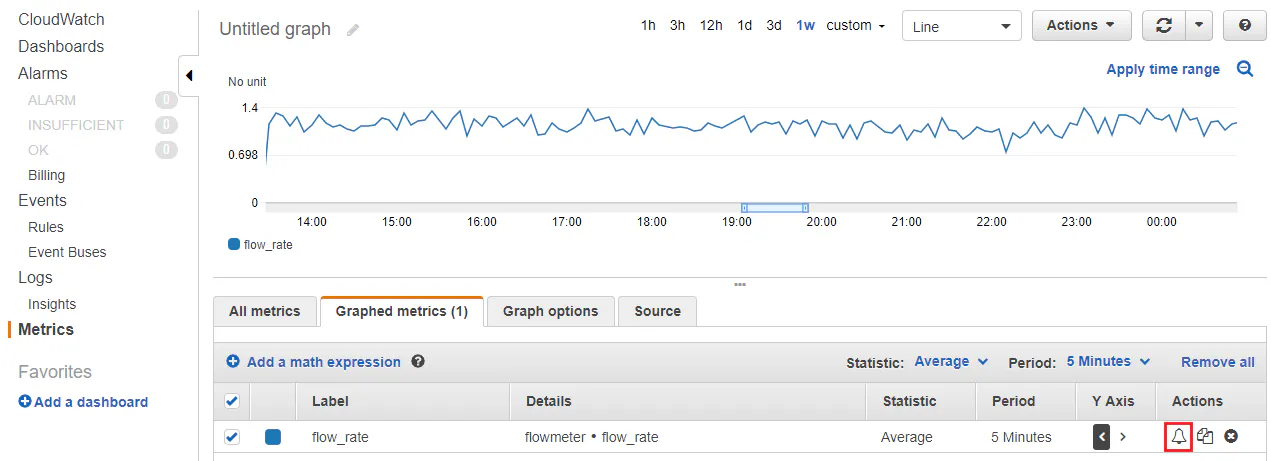“警报详细信息”部分定义度量值何时处于警报状态。对于这个项目，这意味着当 flow_rate 达到一个让用户知道的重要值时。对于此示例，选择了 1.0 的值，但可以通过查看传入数据来确定更实际的数字，以确定正常河流流量生成的流量，并将其设置为高于该数字。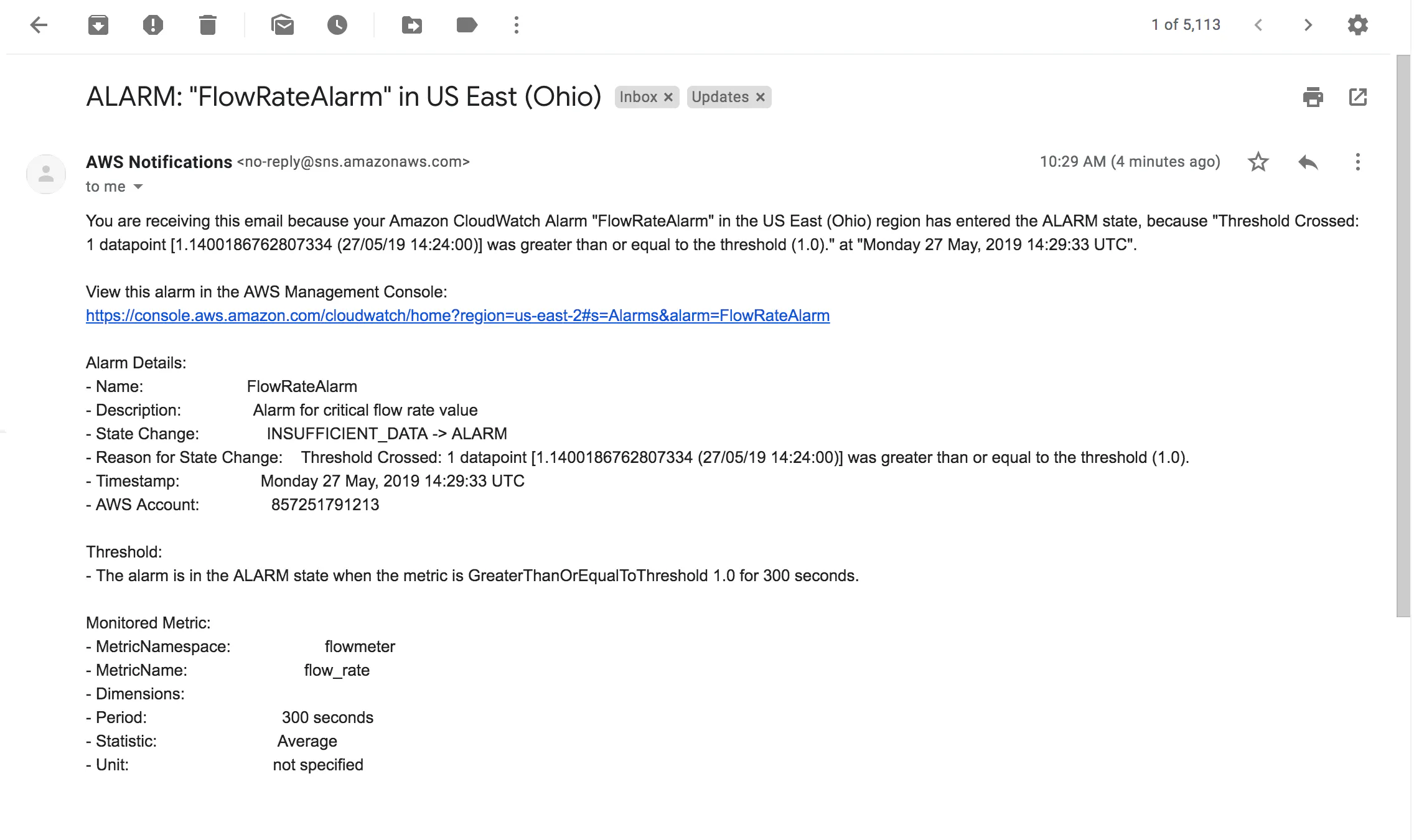加入微信技术交流群关注与非网服务号加入电路城 QQ 交流群